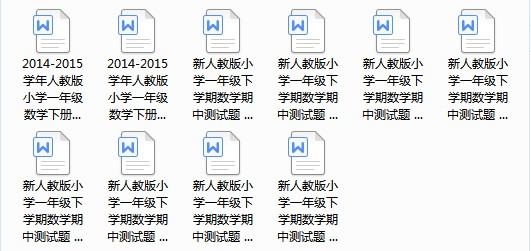• 我会算。（20分）
 12+ 5= 15- 8= 8 + 5= 11- 8= 6+5= 16- 8= 12+ 4= 14- 5= 16- 7= 9-7= 18-10= 9 + 8= 17- 3= 5 + 6= 9+8= 4 +13= 15- 5= 16- 9= 7 + 8= 19- 7=

1、10个一是（     ），（    ）个十是100。

2、一捆铅笔有10枝，5捆再加上8枝是（     ）枝。

3、55这个数，左边的5在（   ）位上，表示（   ）个（   ），右边的5在（    ）位上，表示（   ）个（   ）。